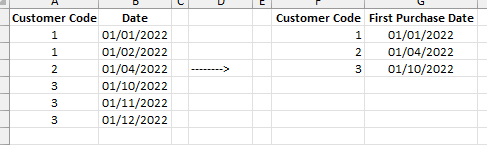cancel
Showing results for
Did you mean:

Fabric is Generally Available. Browse Fabric Presentations. Work towards your Fabric certification with the Cloud Skills Challenge.Helper V

## Calculated column of previous value by date & customerHi guys,
i wish to add a calculated column as in E column above which calculates the previous grade (by date and customer). please note that in my table i have rows which do not hold a grade and presenting blank value.

3 ACCEPTED SOLUTIONSSuper User

We may reduce the number of columns if you have too many. For now please try this

``````Previous Grade =
VAR CurrentDate = 'Table'[Date]
VAR T1 =
CALCULATETABLE ( 'Table', ALLEXCEPT ( 'Table', 'Table'[Customer Code] ) )
VAR T2 =
FILTER ( 'T1', [Date] < CurrentDate )
VAR T3 =
TOPN ( 1, 'T2', [Date] )
VAR T4 =
RETURN
Result``````Super User

``````Previous Date =
VAR CurrentDate = 'Table'[Date]
VAR T1 =
CALCULATETABLE ( 'Table', ALLEXCEPT ( 'Table', 'Table'[Customer Code] ) )
VAR T2 =
FILTER ( 'T1', [Date] < CurrentDate )
VAR T3 =
TOPN ( 1, 'T2', [Date] )
VAR Result =
SELECTCOLUMNS ( 'T3', "@Date", [Date] )
RETURN
Result``````Super User

Try this

``````First Purchase Channel =
CALCULATE (
VALUES ( Data[Purchase Channel] ),
ALLEXCEPT ( Data, Data[Customer Code] ),
Data[Date] = Data[First Purchase Date]
)``````
26 REPLIES 26Super User

Hi @akfir

you can try

``````Previous Grade =
VAR CurrentDate = 'Table'[Date]
VAR T1 =
CALCULATETABLE (
'Table',
ALLEXCEPT ( 'Table', 'Table'[Customer Code] ),
'Table'[Date] < CurrentDate
)
VAR T2 =
TOPN ( 1, 'T1', [Date] )
VAR T3 =
RETURN
Result``````Helper V

there is no RESULT variable to return....Super User
``````Previous Grade =
VAR CurrentDate = 'Table'[Date]
VAR T1 =
CALCULATETABLE (
'Table',
ALLEXCEPT ( 'Table', 'Table'[Customer Code] ),
'Table'[Date] < CurrentDate
)
VAR T2 =
TOPN ( 1, 'T1', [Date] )
VAR Result =
RETURN
Result``````Helper V

<pi>there's not enough memory to complete this operation. please try again later when there may be more memory available.</pi>Super UserHelper V

30K rowsSuper User

We may reduce the number of columns if you have too many. For now please try this

``````Previous Grade =
VAR CurrentDate = 'Table'[Date]
VAR T1 =
CALCULATETABLE ( 'Table', ALLEXCEPT ( 'Table', 'Table'[Customer Code] ) )
VAR T2 =
FILTER ( 'T1', [Date] < CurrentDate )
VAR T3 =
TOPN ( 1, 'T2', [Date] )
VAR T4 =
RETURN
Result``````Helper VWhy is that?Helper V

this is something related to T4, because the previous date column that you helped me as well - still valid and not showing any error.Super User

That means you have multiple records in the same date. Then you can only aggregate by sum or max/min or otherwise provide more filters that can restrict the result to only one record.Helper V

thats maybe because i transferred DATE from date/time to date. what can i do?Super User

You have to restore the date time format in order maitain accuracyHelper V

Perfect thanks! now i wish to add a column of the date referring to this previous value - in my example above - for the first row i want the value to be 01/02/2022Super User

``````Previous Date =
VAR CurrentDate = 'Table'[Date]
VAR T1 =
CALCULATETABLE ( 'Table', ALLEXCEPT ( 'Table', 'Table'[Customer Code] ) )
VAR T2 =
FILTER ( 'T1', [Date] < CurrentDate )
VAR T3 =
TOPN ( 1, 'T2', [Date] )
VAR Result =
SELECTCOLUMNS ( 'T3', "@Date", [Date] )
RETURN
Result``````Helper V

Awsome!! thanks!Super User

@akfir
If my reply solved your problem please consider marking it as an acceptable solution. Thank you and have a great day!Super User

How many columns?Super User

You may also try this

``````Previous Grade =
VAR CurrentDate = 'Table'[Date]
VAR T1 =
CALCULATETABLE ( 'Table', ALLEXCEPT ( 'Table', 'Table'[Customer Code] ) )
VAR T2 =
ADDCOLUMNS ( 'T1', "@Ranking", RANKX ( 'T1', [Date] ) )
VAR T3 =
'T2',
VAR CurrentRank = [@Ranking]
RETURN
LOOKUPVALUE ( [Grade], [@Ranking], CurrentRank + 1 )
)
VAR T4 =
FILTER ( 'T3', [Date] = CurrentDate )
VAR Result =
RETURN
Result``````Helper V

Hi my friend,
can you please help me build a column of the first purchase date by customer? as attached...Super User

Hi @akfir

``````First Purchase Date =
CALCULATE (
MIN ( 'Table'[Date] ),
ALLEXCEPT ( 'Table', 'Table'[Customer Code] )
)``````Announcements#### Power BI Monthly Update - November 2023

Check out the November 2023 Power BI update to learn about new features.#### The largest Power BI and Fabric virtual conference

130+ sessions, 130+ speakers, Product managers, MVPs, and experts. All about Power BI and Fabric. Attend online or watch the recordings.Top Solution Authors
Top Kudoed Authors
Users online (1,617)